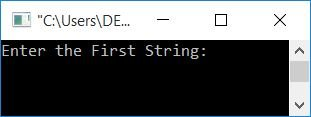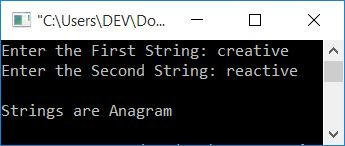# C++ Program to Check Whether Strings are Anagrams or Not

In this article, you will learn and get code to check whether the given two strings by the user are anagrams or not using C++ programming. But before going through the program, let's first understand what an anagram means.

### What are anagram strings?

Two strings will be anagrams of each other if and only if they contain the same number of characters.

Note: The order of the characters doesn't matter.

If two strings are anagrams, then one string can be rearranged to form the other one. For Example:

• abc and cba are anagrams.
• creative and reactive are also anagrams.

Now let's move on to the program.

## In C++, check for anagram strings

To check whether the two strings are anagrams or not in C++ programming, you have to ask the user to enter the two strings to start checking for anagrams and display the result on the screen (whether the string is an anagram or not) as shown here in the following program.

```#include<iostream>
#include<string.h>
using namespace std;
int main()
{
char str1, str2;
int len1, len2, i, j, found=0, not_found=0;
cout<<"Enter the First String: ";
cin>>str1;
cout<<"Enter the Second String: ";
cin>>str2;
len1 = strlen(str1);
len2 = strlen(str2);
if(len1 == len2)
{
for(i=0; i<len1; i++)
{
found = 0;
for(j=0; j<len1; j++)
{
if(str1[i] == str2[j])
{
found = 1;
break;
}
}
if(found == 0)
{
not_found = 1;
break;
}
}
if(not_found == 1)
cout<<"\nStrings are not Anagram";
else
cout<<"\nStrings are Anagram";
}
else
cout<<"\nCharacter count Mismatched!";
cout<<endl;
return 0;
}```

This program was built and runs under the Code::Blocks IDE. Here is its sample run:Now supply any two strings, say creative and reactive, and press the ENTER key to see the following output:First, we must compare the lengths of both strings; if they are equal, we can proceed.Otherwise, print the message with an unequal length, like "Character count mismatched!" Because two strings must be anagrams, they must be the same length.

Now, one by one, compare the first character of the first string to all of the characters of the second string. Then it compares the second character of the first string to all the characters of the second string. Again, compare the third character of the first string to all the characters of the second string, and so on.

While comparing, if any of the characters of the first string get matched to the characters of the second string, then initialize 1 to a variable named "found" and exit the loop. And if there is no match found for any character, then 1 will not initialize to it. So, found will be equal to 0 (its previous or initial value). Therefore, after exiting the inner for loop and checking for the condition if(found==0), it evaluates to be true and 1 gets initialized to not_found, and we exit the outer loop. Therefore, after exiting or completing the outer loop, we have to check whether not_found will be equal to 1 or 0. If not_found is equal to 1, then strings are not anagrams; otherwise, strings are anagrams.

#### Above Program Dry Run

For example, if the user enters two strings as reactive and creative, the dry run of the above program with this input goes like this:

1. The reactive string is set to str1, and the creative string is set to str2 in such a way that:
• str1=r
• str1=e
• str1=a
• str1=c
• str1=t
• str1=i
• str1=v
• str1=e
• str2=c
• str2=r
• str2=e
• str2=a
• str2=t
• str2=i
• str2=v
• str2=e
2. because the length of both strings is 7. Therefore, 7 gets initialized to len1 and len2.
3. Because the lengths are equal, the condition of the if block evaluates to true, and program flow continues inside its block.
4. Using the for loop, 0 gets initialized to i and checks the condition. The condition evaluates to be true, therefore program flow goes inside the loop.
5. 0 gets initialized to found
6. Now, using the for loop, 0 is initialized to j and checked to see if it is less than the value of len1.
7. condition evaluates to be true, program flow goes inside this loop's body.
8. Evaluates the condition str1[i]==str2[j]. Because i and j are both zero, str1==str2 or r==c.
9. condition evaluates to be false, therefore program flow goes to the updation part of this body's first parent loop and increments the value of j.
10. Now j=2
11. Compares str1[i]==str2[j] or str1==str2 or r==r
12. the condition evaluates to true
13. 1 gets initialized to found, and using the break keyword, program flow skips all the next executions of this for loop.
14. After exiting this loop, the value of found is checked to see if it is 0; if it is 0 then the character at the current index of the first string does not match any character of the second string, so 1 is initialized to not_found.
15. After this if statement, another if block is created to check whether the value of not_found is equal to 1 or not. If the condition evaluates to true, then print the string as an anagram; otherwise, print it as an anagram.
16. If it is found, it retains its value of 1, and the program flow proceeds to updating the outer for loop.
17. In this way, now i = 1, found = 0, and j = 0.
18. Compares str1[i]==str2[j], str1==str2, or e==c.
19. Because the condition evaluates to false, compare str1[i] == str2[j] or str1 == str2 or e == r once more.
20. Condition evaluates to false again, so compares str1[i]==str2[j] or str1==str2 or e==e.
21. This time condition evaluates to true, so found=1 and the program flow exits the inner for loop using the break keyword.
22. And begin again at step 14 with the new value of i.
23. Continue the execution until the value of i becomes equal to the value of len1.

#### The same program in different languages

C++ Quiz

« Previous Program Next Program »